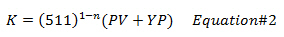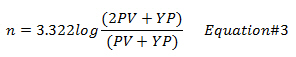## Cutting Carrying Index – Simple Tool To Determine Hole Cleaning

To get the good idea on how good of the hole cleaning is, the another method called Cutting Carrying Index (CCI) is utilized. The CCI is an empirical relationship from real data and the equation is below:

CCI = (K × AV × MW )÷(400,000) — Equation 1

Where;

AV is annular velocity in ft/min.

MW is mud weight in ppg.

K is a Power Law Constant.

The Power Law constant (K) can be calculated from the equation below:Where;

PV is plastic viscosity in centipoises.

YP is yield point in lb/100sqft

n is flow behavior index.

The flow behavior index (n) can be determined by the following equation:Where;

PV is plastic viscosity in centipoises.

YP is yield point in lb/100sqft

How will the CCI tell you about hole cleaning?

If CCI is equal to 0.5 or less, the hole cleaning is poor and the hole problem may be seen.

If CCI is equal to 1.0 or greater, it indicates that the hole cleaning is good.